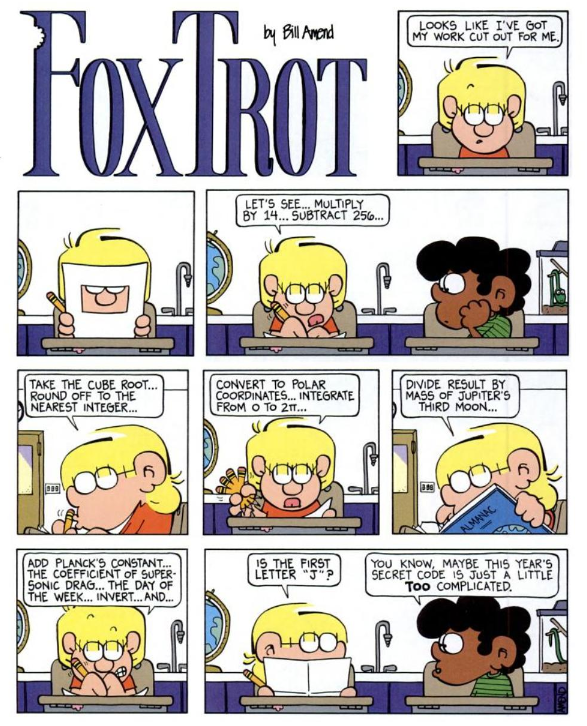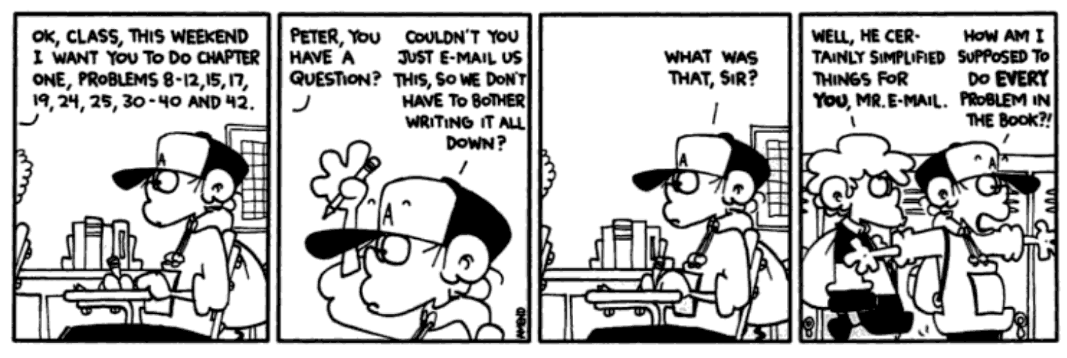# Mathematical Methods - I

Sitabhra Sinha

The great book of Nature lies ever open before our eyes and the true philosophy is written in it ... But we cannot read it unless we have first learned the language and the characters in which it is written ... It is written in mathematical language and the characters are triangles, circles and other geometrical figures.
- Galileo Galilei, 1623 Il Saggiatore (tr. George Polya) p.232

Class Schedule: Tuesday and Thursday (11:30-1:00)7/8/12: Introduction

9/8/12: Basic Stuff

14/8/12: Linear Vector Space

16/8/12: Linear Independence, Basis and Norm

23/8/12: Metric space, Algebra of Linear operators

28/8/12: Algebra of Linear Operators; Angular Momentum; System of masses connected by elastic springs

30/8/12: Inverse operators; Hermitian and Unitary operators

4/9/12: Trace and Determinant of a matrix; Affine transform

6/9/12: Eigenvalues of Hermitian operator; Orthogonalization theorem

11/9/12: Gram-Schmidt orthogonalization; Normal modes; Qualitative theory of 2 coupled first-order differential equations

13/9/12: Using the Jacobian to evaluate stability of solutions of coupled ODEs; Lotka-Volterra equations

18/9/12: Jordan canonical form; Spectral mapping theorem

20/9/12: Infinite-dimensional vector space; Greens function; Hilbert space

25/9/12: Introduction to Complex Analysis: Analytic functions; Mandelbrot and Julia set; Continuity and Derivative of Complex Functions; Cauchys criterion

27/9/12: Mid-term examination

2/10/12: Holiday

4/10/12:

9/10/12: Infinite sequences and series; Convergence tests

11/10/12: Series of functions; Uniform convergence; Weierstrass M-test and Abel test; MacLaurin series; Complex sequence and series

16/10/12: Cauchy's theorem

18/10/12: Cauchy's Theorem in multiply connected region; Morera theorem; Cauchys Integral representation

23/10/12: Local behavior of an analytic function; Analytic contnuation; Taylor series and Laurent series

25/10/12: Classification of singularities; Weierstrass Theorem; Calculus of Residues

30/10/12: Evaluation of integrals using the Residue Theorem

9/11/12: Multi-valued function, branch cuts and Riemann surface

12/11/12: Numerical Solution of differential equations; Integral Transforms: Fourier Transform

13/11/12: Integral Transforms: Laplace Transform and its application to solve differential equations

15/11/12: Theory of Differential Operators and Greens Function

16/11/12: Theory of Greens Function

17/11/12: Greens Function (continued); WKB approximation

29/11/12: End-term examinationAssignments

Textbooks:
Philippe Dennery and Andre Krzywicki: Mathematics for Physicists (Dover, 1996)
George F. Simmons: Differential Equations, with Applications and Historical Notes (Tata McGraw-Hill, 1991) (There is now a new 2007 edition)
Daniel T. Finkbeiner: Introduction to Matrices and Linear Transformations (W H Freeman, 1966)
Harry Hochstadt: The Functions of Mathematical Physics (Dover, 1986)
George B. Arfken, Mathematical Methods for Physicists (Academic Press, 2005)
Jon Mathews and Robert L. Walker: Mathematical Methods of Physics (W A Benjamin, 1970)

Essential web resources for mathematical methods in physics:
James Nearing: Mathematical Tools for Physics